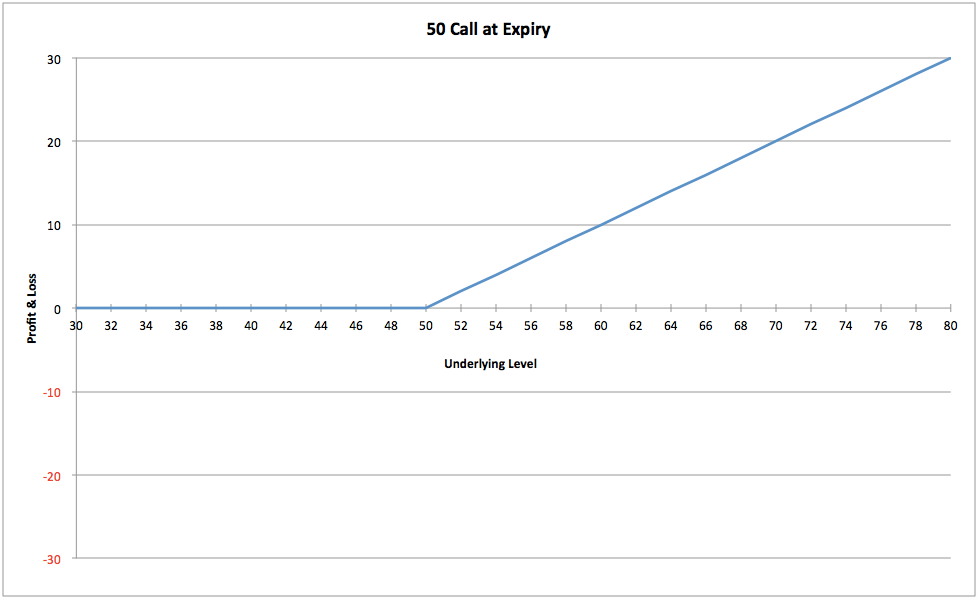top of pageThis publication is part of a series of posts on the Greeks. These posts are a short summary of a part of the content of my book How to Calulate Options Prices and Their Greeks.

The Delta is the first derivative of the value of an option: or the change of the value of the option as compared to the change of the Future or underlying value.

For a call option one can expect the value of that option to increase when the underlying will move up; at the same time a decrease of the value of a put option can be expected. The rate with which the value will increase/ decrease is called the delta. The delta of a call option will have a positive value, the value of the option will increase when the underlying trades at higher levels. The put option, decreasing in value when the underlying moves up, will thus have a negative delta.

The delta is expressed in percentage points (0 to 100% for calls and 0 to -100% for puts), but also quite often in basis points (a 50% delta will be expressed as 0.50 delta). Since the delta is a measure of a portfolio for how long or short one is, the basis points approach will be the most appropriate to value the aggregate position. For instance when owning 100 calls with a 40% delta and having 100 underlying units per option, the aggregate delta in percentage points will be 400,000%. When applying the basis points approach the delta will be 4,000 units, it means that the value of the portfolio will increase by \$ 4,000 for each Dollar that the underlying will trade higher, or one can say as well that he is long 4,000 units.

For a range of options with a certain time to maturity, each strike will have its own delta. When a call option has a delta of 25%, it means that the value of the option would increase by 25 Cts when the underlying moves up 1 Dollar; in fact the call option represents 25% of the total volume of the underlying units.

The combination where one buys the call and sells the put with the same strike and time to maturity, called the Reversal, will result in a delta of 100% (interest rate at 0%, when referring to Stocks; non dividend- paying). The Reversal will have the same P&L distribution as a long position in the underlying (intrinsic value at inception taken into account as well). This implies that when the call option of a certain strike will have a delta of 30% that the put option of that same strike will have a delta of -70%.

Dynamic Delta

Very often one will find charts of call options in books or on the internet looking as below:It is presented as the P&L distribution of the 50 call, however it is never mentioned that the P&L distribution only looks this way at expiry. An option has a life and during its life it will behave differently from when being at maturity.

The chart above shows the 50 call being worthless below 50 and only changing in value above 50. It actually increases by \$ 1 when the underlying is getting \$ 1 more expensive, hence the option has a 0% delta below 50 and a 100% delta above 50, this delta distribution will look as shown below:The former two charts have shown the distribution of the P&L and the Delta at expiry of the option. A very important feature is missing here: i.e. an option has a life and during its life it can already have a value and also have a delta accordingly whilst being far out of the money.

It is actually wrong looking at P&L distributions as if we are dealing with an option at time of expiration. As shown in a previous post (Options Trading, a 4- Dimensional Game NOT a 2- Dimensional one), especially with regards to option combinations, P&L distributions at different times to expiry can look very different from each other.

A 50 call with expiry in a millisecond, and the underlying trading at 40, will have no value and no delta (statistically there is no chance that the call will end up in the money). However when this option will have a long time to maturity (for instance half a year), it will have some value. When looking at the probability distribution for the underlying, depending on volatillity as well, there is some chance that it will expire above 50. This chance will result in a small value for the call, the value of this option will increase when the market moves up and will decrease (towards zero) when the market will come off, hence the option must have a delta. So actually the chart of a call option should look as depicted below:When being half a year to maturity (the green line) the 50 call will start to generate a value, and thus a delta, around the 39 level in the underlying. Around 64 it has gained 100% of delta and the value distribution will now be equal to that one when being at expiry, it moves one on one in comparison with the underlying. When the option has an even longer time to maturity the range in which the option’s delta will increase from 0% to 100% will become much larger (from 34 to 70 in the underlying).

The fact that the delta of a call will grow on the way up, and thus its value increases gradually as depicted above, is sometimes called convexity (because of the convex shape).

In the next publication we will have a look at the impact of time and volatility on the distribution of the delta.

###### Search By Tags
Er zijn nog geen tags.